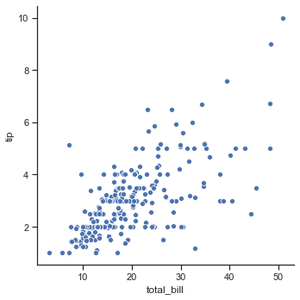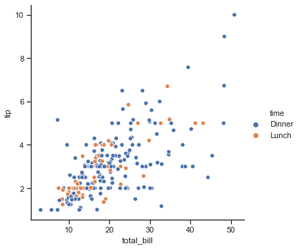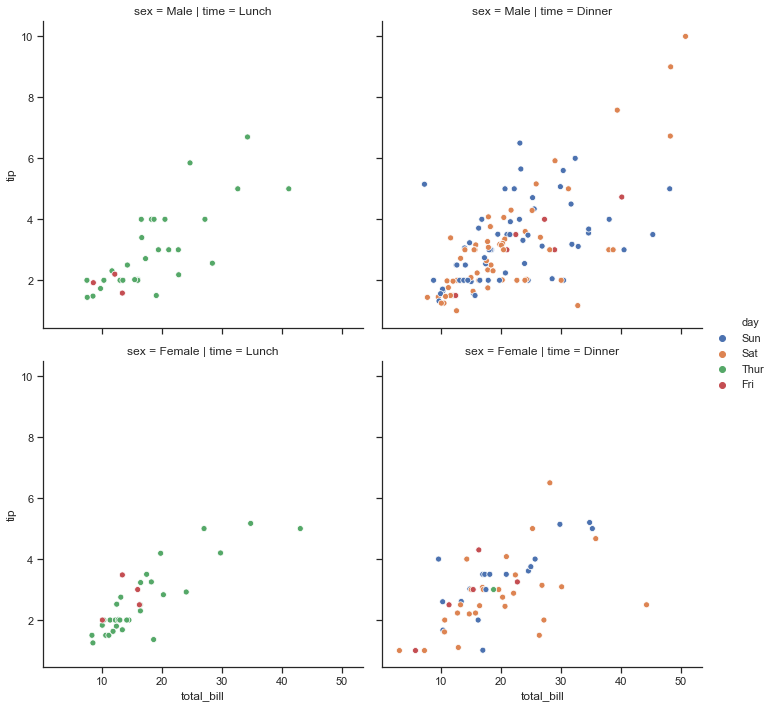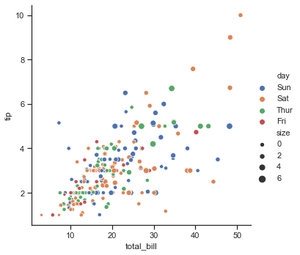# Relational plots in Seaborn – Part I

• Difficulty Level : Easy
• Last Updated : 21 Jul, 2021

Relational plots are used for visualizing the statistical relationship between the data points. Visualization is necessary because it allows the human to see trends and patterns in the data. The process of understanding how the variables in the dataset relate each other and their relationships are termed as Statistical analysis.

Seaborn, unlike to matplotlib, also provides some default datasets. In this article, we will be using a default dataset named ‘tips’. This dataset gives information about people who had food at some restaurant and whether they left tip for waiters or not, their gender and whether they do smoke or not, and more.

Let us have a look to the dataset.

## python3

 `# importing the library` `import` `seaborn as sns`   `# reading the dataset` `data ``=` `sns.load_dataset(``'tips'``)`   `# printing first five entries` `print``(data.head())`

Output :

```   total_bill   tip     sex smoker  day    time  size
0       16.99  1.01  Female     No  Sun  Dinner     2
1       10.34  1.66    Male     No  Sun  Dinner     3
2       21.01  3.50    Male     No  Sun  Dinner     3
3       23.68  3.31    Male     No  Sun  Dinner     2
4       24.59  3.61  Female     No  Sun  Dinner     4```

To draw the relational plots seaborn provides three functions. These are:

• relplot()
• scatterplot()
• lineplot()

## Seaborn.relplot()

This function provides us the access to some other different axes-level functions which shows the relationships between two variables with semantic mappings of subsets.

Syntax :

`seaborn.relplot(x=None, y=None, data=None, **kwargs) `

Parameters :

Example 1: Visualizing the most basic plot to show all the data points in tips dataset.

## Python3

 `# importing the library` `import` `seaborn as sns`   `# selecting style` `sns.``set``(style ``=``"ticks"``)`   `# reading the dataset` `tips ``=` `sns.load_dataset(``'tips'``)`   `# plotting a simple visualization of data points` `sns.relplot(x ``=``"total_bill"``, y ``=``"tip"``, data ``=` `tips)`

Output :Example 2: Grouping data points on the basis of category, here as time.

## Python3

 `# importing the library` `import` `seaborn as sns`   `# selecting style` `sns.``set``(style ``=``"ticks"``)`   `# reading the dataset` `tips ``=` `sns.load_dataset(``'tips'``)`   `sns.relplot(x``=``"total_bill"``,` `            ``y``=``"tip"``,` `            ``hue``=``"time"``,` `            ``data``=``tips)`

Output :Example 3: using time and sex for determining the facet of the grid.

## Python3

 `# importing the library` `import` `seaborn as sns`   `# selecting style` `sns.``set``(style ``=``"ticks"``)`   `# reading the dataset` `tips ``=` `sns.load_dataset(``'tips'``)`   `sns.relplot(x``=``"total_bill"``, ` `            ``y``=``"tip"``,` `            ``hue``=``"day"``,` `            ``col``=``"time"``,` `            ``row``=``"sex"``,` `            ``data``=``tips)`

Output :Example 4: using size attribute, we can see data points having different size.

## Python3

 `# importing the library` `import` `seaborn as sns`   `# selecting style` `sns.``set``(style ``=``"ticks"``)`   `# reading the dataset` `tips ``=` `sns.load_dataset(``'tips'``)`   `sns.relplot(x``=``"total_bill"``, ` `            ``y``=``"tip"``,` `            ``hue``=``"day"``,` `            ``size``=``"size"``,` `            ``data``=``tips)`

Output :My Personal Notes arrow_drop_up
Recommended Articles
Page :